×

Search anything:

# Gaussian blur (filter to blur images)

#### Machine Learning (ML)Get this book -> Problems on Array: For Interviews and Competitive Programming

There was this time I made a pizza and wanted to put a fancy photo of it on social media. So I blurred the background to make it look posher. The one on the left is the original and the one on the right is blurred.This article unfortunately does not examine how to make the pizza, but will ask the other important question - How does blurring the background work?

# Background

## What is a blur filter?

A filter takes an image, processes it and returns an output. In this case, the processing is the blurring.

## What is a Gaussian blur filter, specifically?

Gaussian Filter is one of the most commonly used blur filters in Machine Learning. It employs the technique "kernel convolution".

This filter works by taking a pixel and calculating a value (similar to the mean, but with more bias in the middle). The filter is constructed based on the normal distribution, which is shaped like a bell curve. The idea is that pixels closer to the center pixel have a larger weight and those further a way a smaller weight.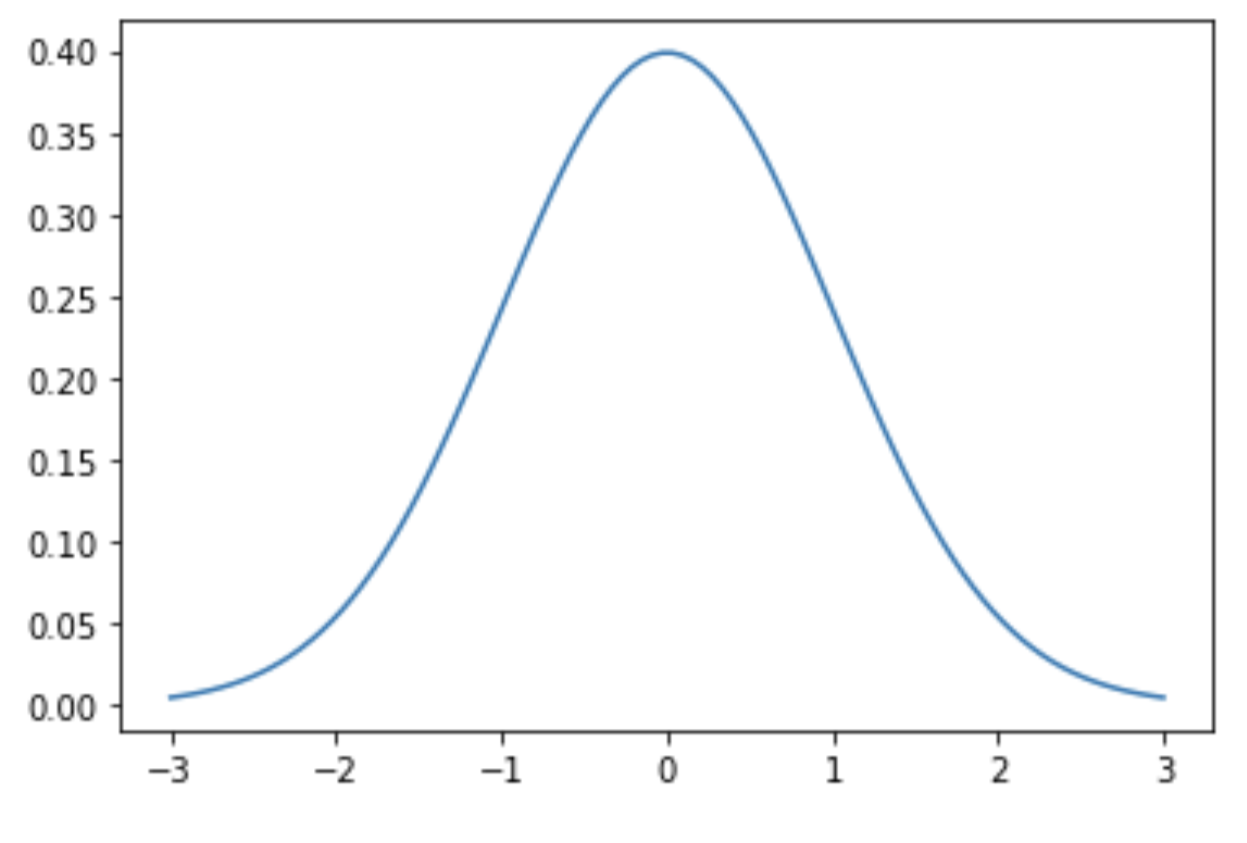This is an example of a normal distribution with mean 0 and standard deviation (and variance, which is standard deviation squared) 1.

A sample Gaussian filter would be as such: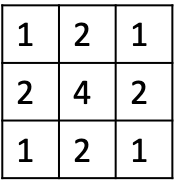Note that the values closer to the middle (in this case represented by 4) are larger than those further away.

Note that the filter has to be an odd number size (e.g. 3x3, 5x5):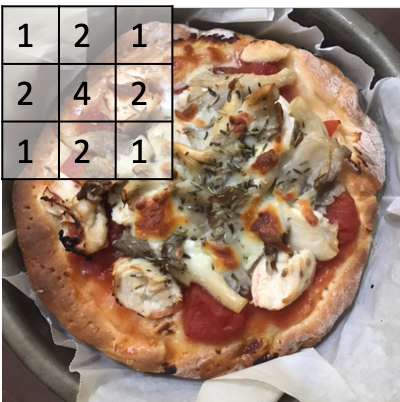This is because the Gaussian filter calculates a value which replaces the number at the middle of the cell, in this case the '4'.

## What are the aspects of a Gaussian blur filter?

One can adjust the standard deviation and the size of the filter to influence the results.

The standard deviation, in other words the average distance from the mean, determines how wide or narrow the bell curve is. The larger the standard deviation, the more wide the bell curve (as generally speaking, the set of numbers is further away from the mean). A larger standard deviation usually also means a larger filter size is necessary, otherwise the change in values in the filter will be huge.

## How long does it take to run the Gaussian blur?

This depends on the size of the image and the filter relative to each other. If the image is large but the filter is small, it is obvious that the processing time will be longer time.

## What is the mean blur and how does the Gaussian blur compare?

The mean blur takes the mean of the pixels instead of using a weighted average like the Gaussian blur. In other words, the mean blur will have a larger blur impact than the Gaussian blur and it will differentiate less between edges.

# Illustrated in examples

Let's take the case where we are no longer working with a pizza, but simply a square with a different coloured corner: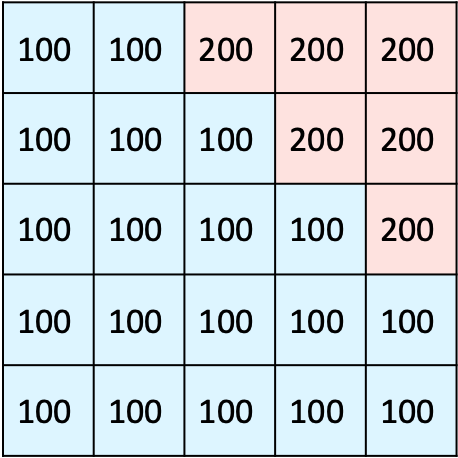We start by placing the filter as such: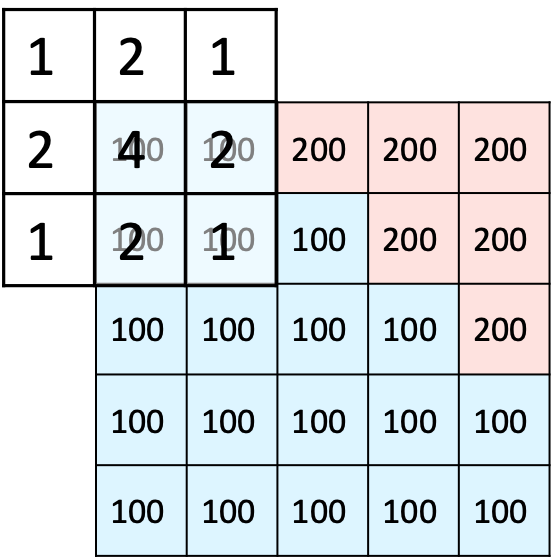By multiplying the grid we get 900. Then, we divide this by the number of pixels, that is 4. So 900 / (4 + 2 + 2 + 1) = 100, this one stayes the same.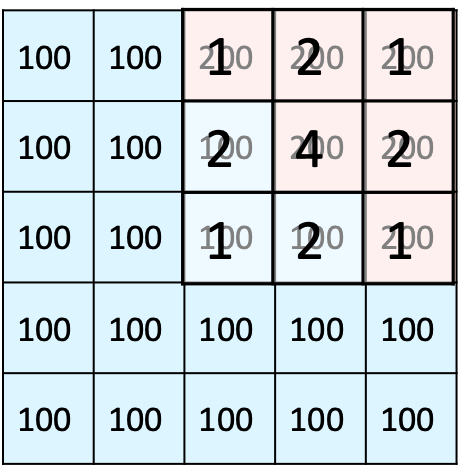A more obvious change would be in this case, where 4 x 200 + 2 x (200 + 200 + 100 + 100) + 1 x (200 + 200 + 200 + 100) = 2700.

We divide this by 16 (the sum of 4, 2 x 4 and 1 x 4) to get 168.75. The colour will be in between blue and pink but more to the pink side. This will likely create a gradient effect and smooth harsh edges.

# Code: how can the Gaussian blur be implemented in Python?

## Step 1: import all the relevant libraries

``````import skimage
from skimage.viewer import ImageViewer
``````

We need skimage to implement the Gaussian blur (this is an inbuilt filter!) and ImageViewer to open the image.

## Step two: import and view the image

Fairly self-explanatory, here we define img to be the image to be read and "test" as a function that opens the image.

``````img = skimage.io.imread(fname="noblur.jpg")
test = ImageViewer(img)
``````

## Step three: created the blurred image

The blurred image is created as a new image, otherwise the calculations will be inaccurate as the numbers keep changing!
Here the skimage.filters.gaussian function takes 3 arguments,

1. img: the image to be modified
2. sigma: this defines the sigma used in the x and y directions
3. truncate: as a real Gaussian is defined from negative to positive infinity, truncate determines the limits of the approx
``````blur = skimage.filters.gaussian(
img, sigma=(10, 10), truncate=3.5, multichannel=True)
``````

Step 4: Check the Image
Launch ImageViewer to see what has happened to the image!

``````viewer = ImageViewer(blurred)
viewer.show()
``````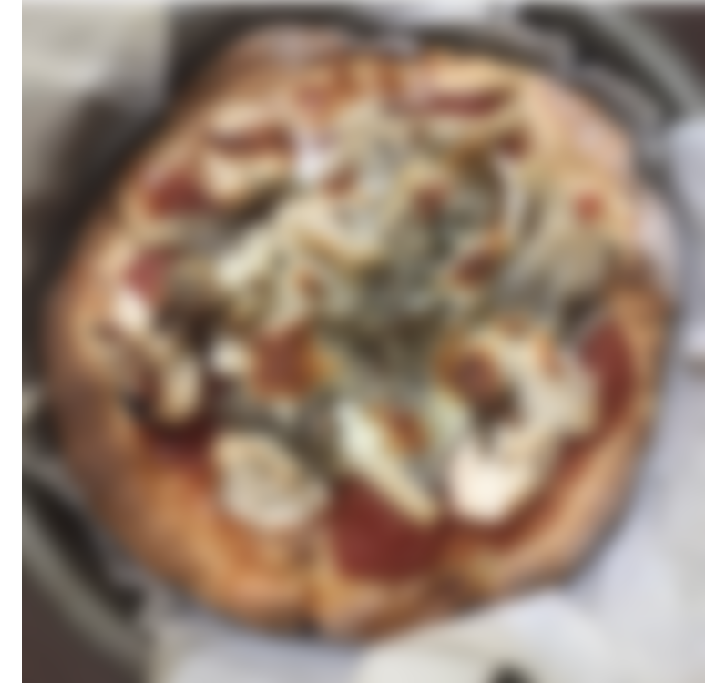The high sigma values yield this pizza - we can still make out that it is a pizza, but barely. High sigma values are preferable if you are not very good at placing your toppings aesthetically and still want to upload the photo to social media. However, if you've burnt your pizza, the blur can't save it because the black will still be there.

# How is the Gaussian blur useful in daily life?

Its main purpose is removing noise from images. This means it is very versatile and can be the base for a photo editing filter for example or just to prepare images for further processing.

# Mini-Conclusion

The Gaussian blur is a type of image processing that applies a filter on an image. This filter takes the surrounding pixels (the number of which is determined by the size of the filter) and returns a single number calculated with a weighted average based on the normal distribution.#### Annie Lee

Economics and Finance | Intern at OpenGenus

Gaussian blur (filter to blur images)